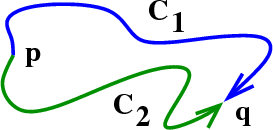# Math Insight

### Image: Two paths between a pair of pointsTwo different paths, $\dlc_1$ and $\dlc_2$, from point $\vc{p}$ to point $\vc{q}$.

Image file: two_paths_between_points.png